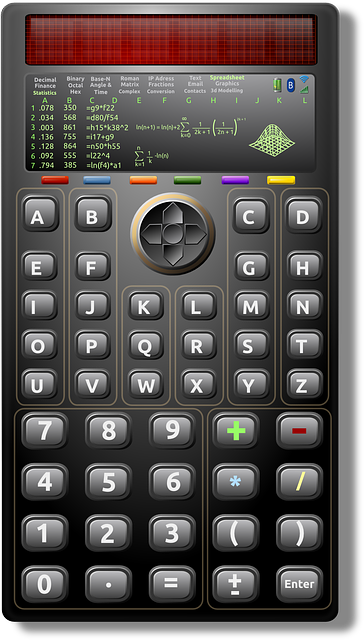# Use of Ti 84 for binomial distributionLet’s talk about the use of the Ti 84 for binomial distribution in this post. The graphic calculator that we refer to is the Ti 84 which most junior colleges in Singapore use for the H2 A Level Math exam.

## Two common functions of Ti 84 for binomial distribution

In the binomial distribution topic in H2 A Level Math Statistics, the two most important functions that we will use from the Ti 84 graphic calculator are:

• binompdf
• binomcdf

## How to find binompdf and binomcdf in Ti 84

To find binompdf and binomcdf, do these:

Step 1: Press [2nd]

Step 2: Press [Vars]. This will bring you to Distr.

Step 3: To go to binompdf, it is [A] for most Ti-84. To go to binomcdf, it is [B] for most Ti 84.

## When do you use binompdf from Ti 84 for binomial distribution questions?

Use binompdf when you are looking at probability of X being a certain value. For example, P(X=3).

## Inputs for binompdf

There are 3 inputs required to use binompdf:

• trials (n)
• p : the probability of success of each trial
• x

## Sample question on use of binompdf in Ti 84

For instance, if X ~ B(10, 0.3), and we are interested in P(X=3), then we will input these into binompdf:

trials = 10; p = 0.3; x= 3

P(X=3) = 0.267 (3 s.f.)

## When do you use binomcdf from Ti 84 for binomial distribution questions?

Use binomcdf when you are looking at probability of X being a certain range. Do note that by default, binomcdf is used to find P(X≤ x). Hence, in order to use binomcdf, make sure you have probability written in terms of P(X≤ x).

For example if you have P(X<3), you’ll have to change it to P(X≤2) in order to use binomcdf.

Similarly, if you have P(X≥ 5), you’ll have to change it to 1- P(X≤4) in order to use binomcdf.

## Inputs for binomcdf

There are 3 inputs required to use binomcdf are the same as binompdf:

• trials (n)
• p : the probability of success of each trial
• x

Key difference is that for binomcdf, we are finding P(X≤x) but for binompdf, we are finding P(X=x).

## Sample question on use of binomcdf in Ti 84

### Sample question 1

For instance, if X ~ B(10, 0.3), and we are interested in P(X≤3), then we will input these into binomcdf:

trials = 10; p = 0.3; x= 3

P(X≤3) = 0.650 (3 s.f.)

### Sample question 2

For instance, if X ~ B(10, 0.3), and we are interested in P(X>5), here are the steps on how to find this probability using Ti-84 binomcdf function.

Since what is required is P(X>5), we have to re-write it in terms of P(X≤x)

P(X>5) = 1- P(X≤5)

Now, we can find P(X≤5) using binomcdf function of Ti-84, with the following inputs:

trials = 10; p = 0.3; x= 5

P(X≤5) = 0.95265

P(X>5) = 1- P(X≤5) = 1 – 0.95265 = 0.473 (3 s.f.)

## A Complete Course on H2 A Level Math Statistics

Want a complete course on H2 A Level Math Statistics?

Check out our course here, where we cover all the topics tested in statistics.

The course includes concepts, types of questions, and how to apply them, so that you’ll score for your exam.

error: Content is protected !!